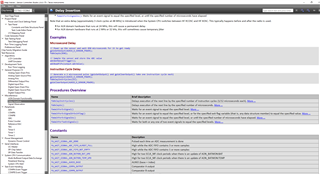If you have a related question, please click the "Ask a related question" button in the top right corner. The newly created question will be automatically linked to this question.

# LAUNCHXL-CC1310: Sensor Controller Studio - timer0Start function - How to calculate time delay.

Part Number: LAUNCHXL-CC1310
Other Parts Discussed in Thread: CC1310

Hi, is there a tool or formula for calculating the delay period based on the MCU, I think the CC1310 is 24Mhz x 2 = 48Mhz CPU clock but I'm not sure. I want to generate a 833 micro/sec and 1sec delay period. Thanks, Steve.

Web page example below:

// Generate timer event after 100 us

`timer0Start(TIMER0_MODE_SINGLE, 2400, 0);`
`gpioSetOutput(AUXIO_O_LED);timer0Start `

Prototype: timer0Start(#mode, mant, #exp)

Sets up AUX Timer 0 to generate events, either one-shot after the specified delay or repeatedly at the specified interval.

Parameter value(s)

• #mode - Select single event or periodical events (TIMER0_MODE_XYZ)
• mant - Delay/interval mantissa value (2-65535). Resulting delay/interval is mant * 2^exp [Sensor Controller clock periods].
• #exp - Delay/interval exponent value (0-15). Resulting delay/interval is mant * 2^exp [Sensor Controller clock periods].

• Sorry Forget to mention the Sensor Controller is using the RTC which I believe is running at 32768 khz.

• Sorry, but I am not sure that I understand what you are asking for? Do you want to insert a delay in your SCS code? If so, you can examples of how this can be done in the Help Viewer:Siri

• Hi, thanks for the reply. Basically I'm trying to understand how the time values is calculated. The following is the example from TI's help page:

// Start an 4.8 ms timeout
timer1Start(TIMER1_MODE_SINGLE, 225, 9);

So my question is how is the value 4.8 microseconds calculated? If the Mantissa is 225 and the exponent is 2^9 the value is 225 x 512 = 115200 microseconds. If the Timer1 is driven by the RTC clock at 4096 hz how is value 4.8 arrived at? Thanks, Steve.

• The timer increments at the Sensor Controller clock rate used in the current power mode.
The sensor controller will run on 2 MHz or 24 MHz, depending on what mode it is in (see the Power and Clock Settings Panel)

The resulting delay/interval is mant * 2^exp [Sensor Controller clock periods]

meaning that if the sensor controller runs on 24 MHz, you will have (225 * 2^9)/24000000 = 4.8 ms

BR

Siri

• Thank you. This now makes sense. Regards, Steve.